[BACK]Computer Modeling in Engineering & SciencesDOI: 10.32604/cmes.2021.014469

ARTICLE

Hybrid Effects of Thermal and Concentration Convection on Peristaltic Flow of Fourth Grade Nanofluids in an Inclined Tapered Channel: Applications of Double-Diffusivity

MCS, National University of Sciences and Technology, Islamabad, Pakistan
*Corresponding Author: Safia Akram. Email: drsafiaakram@gmail.com; drsafiaakram@mcs.edu.pk
Received: 29 September 2020; Accepted: 04 January 2021

Abstract: This article brings into focus the hybrid effects of thermal and concentration convection on peristaltic pumping of fourth grade nanofluids in an inclined tapered channel. First, the brief mathematical modelling of the fourth grade nanofluids is provided along with thermal and concentration convection. The Lubrication method is used to simplify the partial differential equations which are tremendously nonlinear. Further, analytical technique is applied to solve the differential equations that are strongly nonlinear in nature, and exact solutions of temperature, volume fraction of nanoparticles, and concentration are studied. Numerical and graphical findings manifest the influence of various physical flow-quantity parameters. It is observed that the nanoparticle fraction decreases because of the increasing values of Brownian motion parameter and Dufour parameter, whereas the behaviour of nanoparticle fraction is quite opposite for thermophoresis parameter. It is also noted that the temperature profile decreases with increasing Brownian motion parameter values and rises with Dufour parameter values. Moreover, the concentration profile ascends with increasing thermophoresis parameter and Soret parameter values.

Keywords: Nanofluids; thermal and concentration convection; peristaltic flow; inclined tapered channel; fourth grade fluid

1  Introduction

Fluid transport with the help of peristaltic waves is first studied by Latham . Since then it has been the central domain of research interest in physiological and mechanical situation. Peristaltic pumping is a mean or device for pumping fluids. It carries the fluid from lower pressure to higher pressure along the tube through contraction wave. This process occurs in many physiological mechanisms. For example, food movement from esophagus via stomach to intestine, urine excretion of a bladder through kidneys, movement of sperms and ova in male and female (fallopian tube) reproductive system respectively and chyme movement of gastrointestinal tract. Furthermore, peristaltic action relates to lump transfer in lymphatic vessels, blood flow in minute arteries and veins, and bile conduct through bile duct. There are many practical utilities of peristaltic pumping in biomechanical systems. Moreover, to pump any corroding material, roller and finger pumps are utilized to avoid the direct contact with the surface. The initial mathematical models of peristalsis were presented by Shapiro et al.  and Fung et al. . They acquired it through their working on a sinusoidal wave in endlessly long symmetrical channel or tube. In later studies, the focus was to further explore the peristaltic action for Newtonian and non-Newtonian fluids in diverse situations. Numerous experimental, analytical, and numerical models have been discussed in this regard. Though much work is available on the subject but few recent research are mentioned by the studies given at .

The field of practical application of nanofluids in industry and engineering has renowned the interest of the researchers. These applications are related to photodynamic therapy, the lotus effect for self-cleaning surfaces, primary cellular level of biological organisms, membranes for filtering on size or charge (e.g., for desalination), shrimps snapping along with beetle wings super-hydrophobic process, use of charged polymers for lubrication, nano porous materials for size exclusion chromatography, molecular motors, drug transfer, neuro electronic interfaces, cancer diagnostics and therapies, protein engineering, machines for cell repair and light casting on molecular motor cells such as kinesis and charged filtration in the kidney basal membrane, etc. . Choi et al.  coined the word nanofluid which designates to the fluid containing nano-sized particles for conventional heat transfer. Moreover, the liquid contains ultrafine particles which are below 50 nm diameter. These particles could be deducted with metals like (Cu, Al), nitrides (SiN), oxides (Al2O2), or in non-metals namely nanofibers, graphite, carbon nanotubes and droplets. Masuda et al.  propagated that nanofluids can be used in advanced nuclear system because they have the characteristics to enhance the thermal conductivity. An analytical model was followed by Buongiorno et al. [14,15] which is based on the nanofluids flow. The model implied convective transport in nanofluids with Brownian diffusion and thermophoresis. It is revealed in his studies that Brownian diffusion and thermophoresis were significant nanoparticle or base-fluid slip process. This also explains abnormal convective heat transfer enhancement in nanofluids. The concept of nanofluids both in peristaltic and non-peristaltic flow are mentioned in .

A fluid dynamics phenomenon, termed as double diffusive convection, is a convection directed by two different density gradients having different rates of diffusion. Fluids convection is propelled by density variation under the influence of gravity. Such density variation can occur due to the gradients in fluid composition or differences in temperature through thermal expansion. Compositional and thermal gradients usually diffuse over time which hampers the ability to conduct the convection. Therefore, that gradient requires in the flow areas to carry on convection. We can find out an example of double diffusive convection in oceanography. Here salt and heat concentration dwell with various gradients and diffuse at varying rates. Influence of cold water, such as from iceberg, can affect these variables. Akbar et al.  has examined peristaltic flow in nanofluid with double diffusive natural convection. Further theoretical works on double diffusion are mentioned in .

Limited work has been traced in literature review on inclined tapered channel with double diffusive convection on peristaltic flow of nanofluids. Hence, inclined tapered channel on peristalsis is considered with double diffusive convection flow for the current study by taking non-Newtonian nanofluid.

2  Formulation and Methodology

Let’s consider the fourth-grade peristaltic transport in tapered channel with 2d width. The sinusoidal wave propagates at constant velocity c along channel walls. At upper and lower walls the temperature, solute and concentration of nanoparticles is T0,C0,Θ0 and T1,C1,Θ1 respectively. In addition, we also assume that the channel is inclined at an angle α. For 2-dimensional and directional flow the field of velocity is characterized as W̃=(Ũ(X̃,,t̃),(X̃,,t̃),0). The flow geometry of the physical model is now described in Fig. 1. The walls of tapered channel that are at lower level H̃1 and upper level H̃2 are represented in a fixed frame of reference as

H̃1(X̃,t̃)=-d-m̃X̃-ã1sin[2πλ(X̃-ct̃)+φ],H̃2(X̃,t̃)=d+m̃X̃+ã2sin[2πλ(X̃-ct̃)],(1)

here t̃,c,λ, (ã1,ã2) and m̃ (m̃<<1) refers to time, velocity propagation, wavelength, lower and upper walls amplitudes and parameter of non-uniform tapered channel, respectively. The phase difference ϕ lies within the range of 0φπ,φ=0 is symmetrical channel with out-of-phase waves, i.e., the two walls move at the same speed. Also a1̃,a2̃,d, and ϕ fulfil the condition ã12+ã22+2ã1ã2cosφ(2d)2) .

Against fourth grade fluid, the stress tensor is described by 

τ=-PI+S,S=μÃ1+α̃1Ã2+α̃2Ã12+β̃1Ã3+β̃2(Ã1Ã2+Ã2Ã1)+β̃3(tracÃ12)Ã1+γ̃1Ã4+γ̃2(Ã3Ã1+Ã1Ã3)(2)

+γ̃3Ã22+γ̃4(Ã12Ã2+Ã2Ã12)+γ̃5trac(Ã2)Ã2+γ̃6trac(Ã2)Ã12+(γ̃7tracÃ3+γ̃8tracÃ2Ã1)Ã1,(3)

Ã1=(V)+(V)T̃,(4)

Ãi=dÃi-1dt+Ãi-1(V)+(V)i-1T̃Ã,(5)

where μ refers to constant viscosity, α̃1,α̃2,β̃1-β̃3,γ̃1-γ̃8 refers for material constants, T̃ refers to transpose and Ãi denotes Rivilin–Ericksen tensors.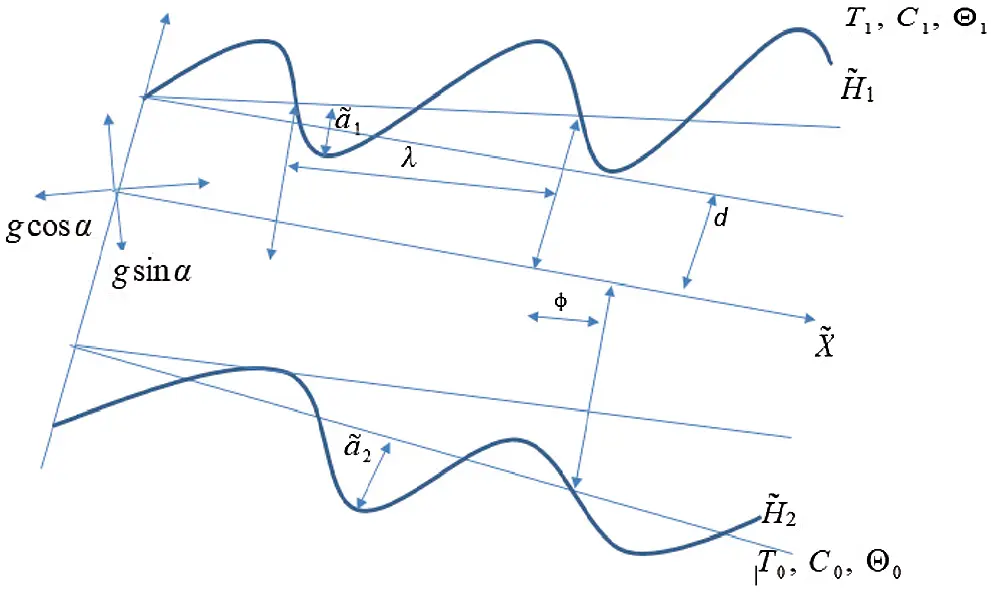Figure 1: Flow geometry of the physical model

The equation of continuity, momentum, temperature, fraction of nanoparticles and the solute concentration of an incompressible fluid for two-dimensional cases is given as

ŨX̃+=0,ρf(Ũt̃+ŬŨX̃+Ũ)=-PX̃+X̃(SX̃X̃)+(SX̃)+ρgsinα(6)

+g{(1-Θ0)ρf0{βT(T-T0)+βC(C-C0)}-(ρp-ρf0)(Θ-Θ0)},(7)

ρf(t̃+ŬX̃+)=-P+X̃(SX̃)+(S̃)-ρgcosα,(ρc)f(t̃+ŬX̃+)T=ε(2TX̃2+2T2)+(ρc)p{DB(ΘX̃TX̃+ΘT)(8)

(DTT0)[(TX̃)2+(T)2]}+DTC(2CX̃2+2C2),(9)

(t̃+ŬX̃+)C=Ds(2CX̃2+2C2)+DTC(2TX̃2+2T2),(10)

(t̃+ŬX̃+)Θ=DB(2ΘX̃2+2Θ2)+(DTT0)(2TX̃2+2T2),(11)

In the above equations ρf,g,ρf0,ρp,T,C,Θ,DB,DT,DCT,Ds,DTC,βC,βT,ε,(ρc)p,(ρc)f refers to base fluid density, gravity acceleration, fluid density at T0, particles density, temperature, concentration, nanoparticle volume fraction, Brownian diffusion coefficient, thermophoretic diffusion coefficient, soret diffusively, solutal diffusively, Dufour diffusively, volumetrically solutal expansion coefficient of a fluid, volumetrically thermal expansion coefficient of a fluid, thermal conductivity, nanoparticle heat capacity and fluid heat capacity, respectively.

Defining the subsequent dimensionless quantities

u=Ũc,v=c,x=X̃λ,y=d,δ=dλ,p=d2pμcλ,t=ct̃λ,h1=H̃1d,h2=H̃2d,a=ã1d,b=ã2d,m=m̃λd,Re=ρfcdμ,Pr=(ρc)fυε,θ=T-T0T1-T0,γ=C-C0C1-C0,Le=υDs,Ω=Θ-Θ0Θ1-Θ0,Ln=υDB,NCT=DCT(T1-T0)(C1-C0)Ds,NTC=DCT(C1-C0)ε(T1-T0),Nb=(ρc)pDB(Θ1-Θ0)ε,Nt=(ρc)pDT(T1-T0)εT0,GrF=g(ρp-ρf)(Θ1-Θ0)d2μ0c,Grt=gd2(1-Θ0)(T1-T0)ρfβTμ0c,Grc=g(1-Θ0)ρfβc(C1-C0)d2μ0c,λ̃n=α̃icμb0(i=1,2),κ̃i=β̃ic2μb02(i=1,2,3),η̃i=γ̃nc3μb03(i=1-8)Sij=dμS̃ij,(i=1,2,3),u=ψy,v=-δψx.(12)

In above dimensionless quantities Pr , δ,Re,Grc,GrF,GrT,Le,Nb,Ln,Nt,NCT,NTC,θ,Ω and γ representing Prandtl number, wave number, Reynolds number, solutal Grashof number, nanoparticle Grashof number, thermal Grashof number, Lewis number, parameter of Brownian motion, nanofluid Lewis number, parameter of thermophoresis, parameter of Soret, parameter of Dufour, dimensionless temperature, solutal concentration and fraction nanoparticle, respectively.

By using Eq. (6), Eq. (13) is automatically satisfied and Eqs. (7)(12) for stream function ψ, temperature θ, nanoparticle fraction γ and solute concentration Ω in wave frame becomes

Reδ(ψtyψyψxy-ψxψyy)=-px+δx(Sxx)+y(Sxy)+ReFrsinα+Grtθ+Grcγ-GrFΩ,(13)

Reδ3(ψtxψxψxy-ψyψxx)=-pmy+δ2x(Syx)+δy(Syy)-δReFrcosα+δ(Grtθ+Grcγ-GrFΩ),RePrδ(θt+ψyθx-ψxθy)=(θyy+δ2θxx)+NTC(δ2γxx+γyy)+Nb(δ2Ωxθx+θyΩy)(14)

+Nt(δ2(θx)2+(θy)2),(15)

ReδLe(γt+ψyγx-ψxγy)=(δ2γxx+γyy)+NCT(δ2θxx+θyy),(16)

ReδLn(Ωt+Ωxψy-ψxΩy)=(δ2Ωxx+Ωyy)+NtNb(δ2θxx+θyy),(17)

Now using supposition of long wavelength and low number of Reynolds, the Eqs. (13)(17) becomes

0=-px+ySxy+ReFrsinα+Grtθ+Grcγ-GrFΩ,(18)

0=-py,(19)

2θy2+NTC2γy2+Nb(θyΩy)+Nt(θy)2=0,(20)

2γy2+NCT2θy2=0,(21)

2Ωy2+NtNb2θy2=0,(22)

Eliminate pressure from Eqs. (18) and (19) we get

2y2Sxy+Grtθy+Grcγy-GrFΩy=0,(23)

where

Sxy=2ψy2+2Γ(2ψy2)3,(24)

and Γ=κ2+κ3 is Deborah number.

In wave frame the boundary conditions regarding stream function Ψ , temperature θ, fraction of nanoparticle Ω and solute concentration γ are described as:

ψ=-F2at y=h1=-1-mx-asin[2π(x-t)+φ],ψ=F2at y=h2=1+mx+bsin[2π(x-t)],(25)

ψy=0at y=h1=-1-mx-asin[2π(x-t)+φ],

ψy=0at y=h2=1+mx+bsin[2π(x-t)],(26)

θ=0,at y=h1 and θ=1,at y=h2,(27)

Ω=0,at y=h1,andΩ=1,at y=h2,(28)

γ=0,at y=h1, and γ=1,at y=h2,(29)

3  Exact Solution

The exact solution of the volume fraction of nanoparticles which satisfies the relevant condition (28) is described as

Ω=(y-h1)(NtNb+1)h2-h1-Nt(e-ξy-e-ξh1)Nb(e-ξh2-e-ξh1),(30)

The exact solution of the solutal (species) concentration which satisfies the relevant condition (29) is described as

γ=(NCT+1)(y-h1)h2-h1-NCT(e-ξy-e-ξh1)e-ξh2-e-ξh1,(31)

The exact solution of temperature which satisfies the relevant condition (27) is described as

θ=e-ξy-e-ξh1e-ξh2-e-ξh1,(32)

where

ξ=Nb+Nt(h2-h1)(1-NCTNTC),(33)

4  Analytical Technique

The differential Eqs. (19) and (24) are non-linear so it is difficult to find exact solutions to these equations. So regular perturbation technique is used for finding the solutions of Eqs. (19) and (24). Expand now Ψ,p and F as

ψ=ψ0+Γ(ψ1),(34)

p=p0+Γ(p1),(35)

F=F0+Γ(F1).(36)

With assistance from Eqs. (34)(36) into Eqs. (19), (24) and (26) combining like powers of Γ, we get the following system as follows:

System of order Γ0

4ψ0y4=-(Grtθy+Grcγy-GrFΩy),(37)

p0x=3Ψ0y3+ReFrsinα+Grtθ+Grcγ-GrFΩ,(38)

ψ0=-F02at y=h1=-1-mx-asin[2π(x-t)+φ],(39)

ψ0=F02at y=h2=1+mx+bsin[2π(x-t)],(40)

ψ0y=0at y=h1=-1-mx-asin[2π(x-t)+φ],(41)

ψ0y=0at y=h2=1+mx+bsin[2π(x-t)],(42)

System of order Γ1

4ψ1y4=-22y2(2ψ0y2)3,(43)

p1x=3Ψ1y3+2y(2ψ0y2)3,(44)

ψ1=-F12at y=h1=-1-mx-asin[2π(x-t)+φ],(45)

ψ1=F12at y=h2=1+mx+bsin[2π(x-t)],(46)

ψ1y=0at y=h1=-1-mx-asin[2π(x-t)+φ],(47)

ψ1y=0at y=h2=1+mx+bsin[2π(x-t)],(48)

4.1 Solution for Zeroth Order System

Solution of Eq. (37) that satisfies the boundary conditions (39)(42) is described as follows:

ψ0=L4y3+L3y2+L2y+L1+ξ0y424-ξ1sinh(ξy)ξ4+ξ1cosh(ξy)ξ4,(49)

The pressure gradient for this order is described as

p0x=ReFrsinα+ξ1sinh(ξy)ξξ1cosh(ξy)ξ+Grt(eξyeh1ξeh2ξeh1ξ)+ξ0y+6L4   +Grc((NCT+1)(yh1)h2h1NCT(eξyeh1ξ)eh2ξeh1ξ)   GrF((yh1)(NtNb+1)h2h1Nt(eξyeh1ξ)Nb(eh2ξeh1ξ)), (50)

where ξi,s constants are used for simplifying equations and are described in Appendix. The remaining constants L1, L2, L3 and L4 are determined using boundary conditions (39)(42) and are described in Appendix.

4.2 Solution for First Order System

Using solution zero-order (49) into (43), the solution of Eq. (43) which satisfies the boundary conditions (45)(48) is described as follows:

ψ1=-314L4ξ02y7+ξ0ξ8y6240L3+L8y3+L7y2+L6y+L5-1224ξ03y8+ξ9y5120+ξ11y3e-ξy+124y4(ξ8-36ξ02ξ1e-ξyξ4)+y2(ξ12e-ξy-3ξ0ξ12e-2ξy4ξ6)+y(ξ13e-ξy+ξ14e-2ξy)-2ξ13e-3ξy9ξ8+ξ10e-2ξy+ξ15e-ξy,(51)

The pressure gradient for this order is described as

p1x=-45L4ξ02y4+ξ0ξ8y32L3+6L8-123ξ03y5+3ξ02ξ1y4e-ξy2ξ-ξ3ξ11y3e-ξy+ξ9y22-18ξ02ξ1y3e-ξyξ2+54ξ02ξ1y2e-ξyξ3+y2(6ξ0ξ12e-2ξyξ3-ξ3ξ12e-ξy)+6ξ13e-3ξyξ5+6(3ξ0ξ12e-2ξy2ξ5-ξξ12e-ξy)+y(ξ8-36ξ02ξ1e-ξyξ4)+9ξ2ξ11y2e-ξy+y(ξ13ξ3(-e-ξy)-8ξ14ξ3e-2ξy)-8ξ3ξ10e-2ξy-ξ3ξ15e-ξy+3ξ2ξ13e-ξy+12ξ2ξ14e-2ξy+6y(ξ2ξ12e-ξy-3ξ0ξ12e-2ξyξ4)-18ξξ11ye-ξy+6ξ11e-ξy+6(6L4+ξ0y+ξ1ξ(sinh(ξy)-cosh(ξy)))(6L4y+2L3+ξ0y22+ξ1ξ2(cosh(ξy)-sinh(ξy)))2,(52)

where ξi,s constants are used for simplifying equations and are described in Appendix. The remaining constants L5, L6, L7 and L8 are determined using boundary conditions (45)(48) and are described in Appendix.

Now for small parameter Γ, summarizing the perturbation results we have

ψ=ψ0+Γψ1,(53)

px=p0x+Γp1x,(54)

Δp=Δp0+ΓΔp1,(55)

Defining F=F0+ΓF1 and using F0=F-ΓF1 and then ignoring terms larger than O(Γ) the results obtained by Eq. (53) to Eq. (55) showing up till Γ.

For average pressure increase the non-dimensional expression is given as follows:

Δp=0101pxdxdt.(56)

5  Different Wave Forms

The expression (in non-dimensional form) for the considered wave forms is defined as follows:

1. Multisinusoidal wave

h1(x)=-1-mx-asin[2nπ(x-t)+φ],h2(x)=1+mx+bsin[2πn(x-t)].

2. Triangular wave

h1(x)=-1-mx-a[8π3i=1(-1)i+1(2i-1)2sin(2π(2i-1)(x-t)+φ)],

h2(x)=1+mx+b[8π3i=1(-1)i+1(2i-1)2sin(2π(2i-1)(x-t))].

3. Trapezoidal wave

h1(x)=1mxa[32π2i=1sinπ8(2i1)(2i1)2sin(2π(2i1)(xt)+φ)], h2(x)=1+mx+b[32π2i=1sinπ8(2i1)(2i1)2sin(2π(2i1)(xt))].

6  Graphical Outcomes

To interpret the results quantitatively we consider the instantaneous volume flow rate F(x,t) periodic in (xt)  asF(x,t)=Q+asin[2π(x-t)+φ]+bsin[2π(x-t)] here Q is average time of flow through a single wave cycle and F=h1h2udy.

To observe the graphical outcomes of concentration, temperature, nanoparticle fraction, pressure gradient, pressure rise and streamlines Figs. 211 are displayed. The temperature profile effect is plotted for the different values of Nb and NTC in Figs. 2a and 2b. It is seen in Fig. 2a that temperature profile behaviour decreases with increasing Nb values. This is because temperature exhibits a direct relationship with Nb. In Fig. 2b the temperature profile show opposite effect as compared with Nb. Here temperature effects increases with increasing NTC values. Fig. 3 shows the impact of Nt and NCT on concentration profile. It is shown in Figs. 3a and 3b that concentration profile increases with increasing Nt and NCT values. This is due to the direct relationship of concentration with Nt and NCT. To view the impact of nanoparticle fraction on Nb, Nt and NTC Figs. 4a4c are plotted. It is shown in Fig. 4 that behavior of nanoparticle fraction decreases because of the increasing values of Nb and NTC (see Figs. 4a and 4c), whereas the behaviour of nanoparticle fraction is quite opposite for Nt. In this case nanoparticle fraction increases due to the increasing Nt values.Figure 2: (a) Profile of temperature (θ) for various Nb values (sinusoidal wave). (b) Temperature (θ) profile for various NTC values (sinusoidal wave)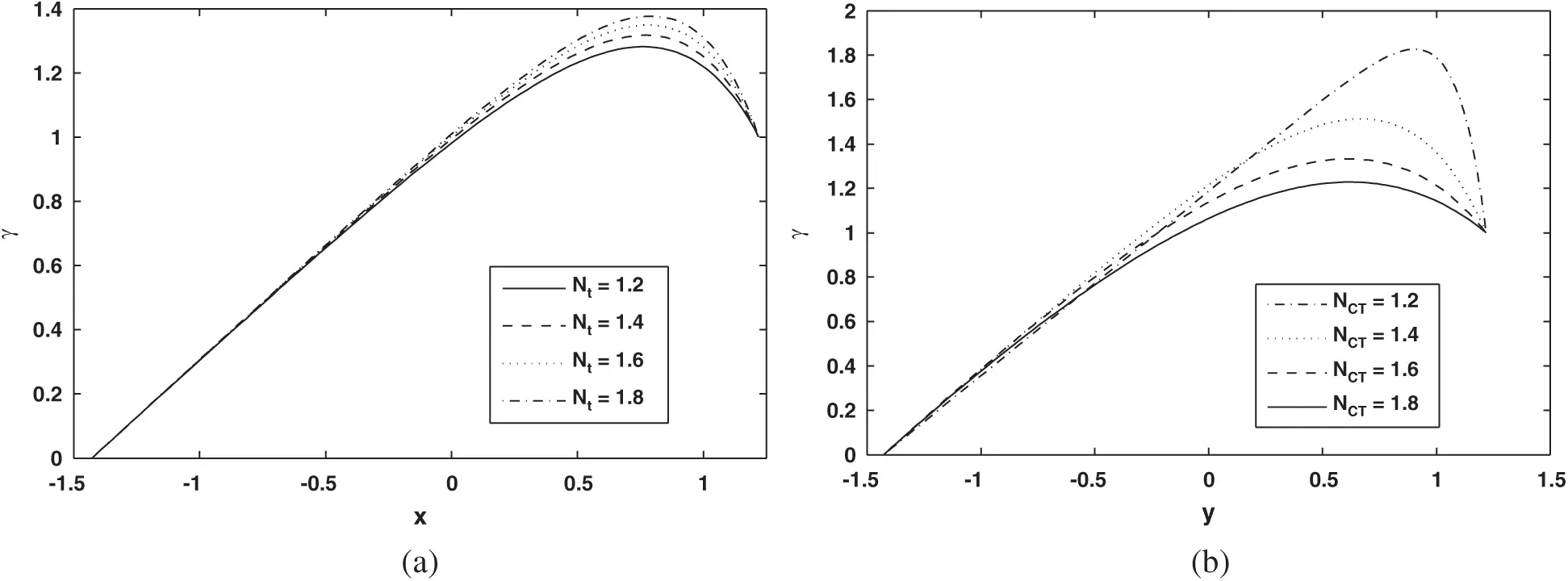Figure 3: (a) Profile of Solutal concentration (γ) for various Nt values (sinusoidal wave). (b) Solutal concentration (γ) profile for various NCT values (sinusoidal wave)Figure 4: (a) Profile of Nanoparticle fraction (Ω) for various Nb values (sinusoidal wave). (b) Nanoparticle fraction (Ω) profile for various Nt values (sinusoidal wave). (c) Nanoparticle fraction (Ω) profile for various NTC values (sinusoidal wave)

To study the graphical results of pressure, rise Figs. 5a5d are plotted. As it turns out in Figs. 5a and 5b that pressure rise decreases within the regions where Δp>0,Q<0 (retrograde pumping), Δp>0,Q>0 (peristaltic pumping) and Δp=0 (free pumping), whereas behavior is opposite over the regions where Δp<0,Q>0 (copumping region). Here pressure rise enhanced by increasing values of m and Γ. Figs. 5c and 5d show the pressure rise actions for the various Re and Nt values. From these figures we can see that pressure rise increases in all pumping regions (peristaltic, retrograde, copumping and free) by increasing values of Re and Nt. Graphical behavior of pressure gradient is illustrated in Fig. 6a6c. It is represented in Fig. 6a that when xε[0,0.7] the pressure gradient decreases by increasing Nb values, while the pressure gradient behaviour is quite opposite when xε[0.7,1]. Here pressure gradient increases because of increasing values of Nb. Fig. 6b indicates that when xε[0.7,1] the value of the pressure gradient decreases because of increasing values of Γ. It shows up in Fig. 6c that the behavior of pressure gradient decreases due to the increasing values of Fr. The pressure gradient behaviour for the various wave forms is shown in Figs. 7a7d. From these figures it is seen that trapezoidal waves are found to have maximum pressure gradient.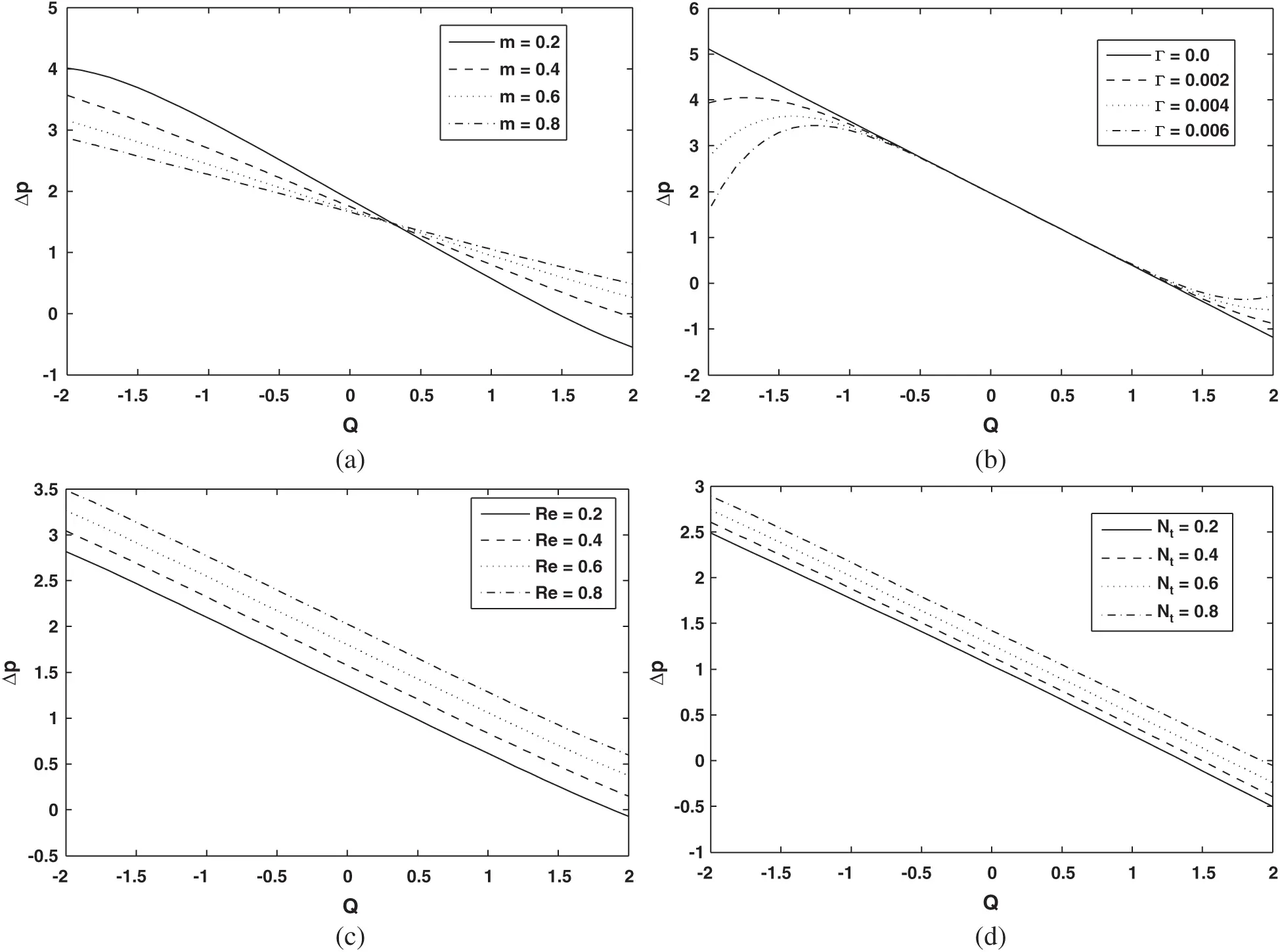Figure 5: (a) Pressure rise over one wavelength (Δp) against volume flow rate (Q) for various m values (sinusoidal wave). (b) Pressure rise over one wavelength (Δp) against volume flow rate (Q) for various Γ values (sinusoidal wave). (c) Pressure rise over one wavelength (Δp) against volume flow rate (Q) for various Re values (sinusoidal wave). (d) Pressure rise over one wavelength (Δp) against volume flow rate (Q) for various Nt values (sinusoidal wave)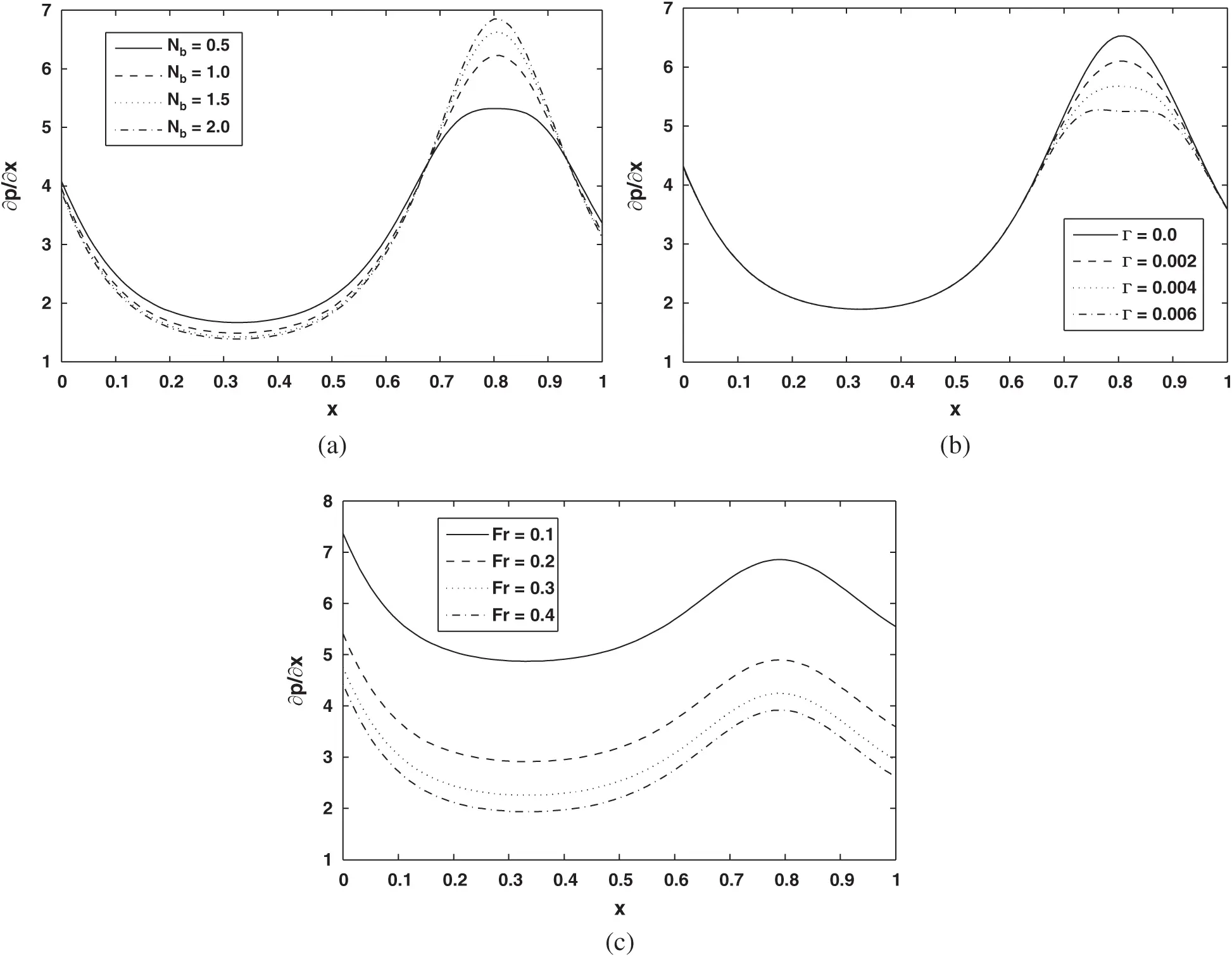Figure 6: (a) Pressure gradient (p/x) against axial distance (x) for various Nb values (sinusoidal wave). (b) Pressure gradient (p/x) against axial distance (x) for various Γ values (sinusoidal wave). (c) Pressure gradient (p/x) against axial distance (x) for various Fr values (sinusoidal wave)Figure 7: (a–d): Pressure gradient (dp/dx) against axial distance (x) for different wave shapes

For studying the phenomenon of trapping Figs. 811 are plotted. Streamlines for the different values of NCT and m are displayed in Figs. 8 and 9. It shows up in Figs. 8 and 9 that the size and number of the trapped bolus are increased by rising values of NCT and m. Fig. 10 is plotted to observe the streamlines pattern of the streamlines for different Nb values. Number of trapping bolus is observed to decrease by increasing Nb values. Patterns of streamlines for various wave types are shown in Figs. 11a and 11b.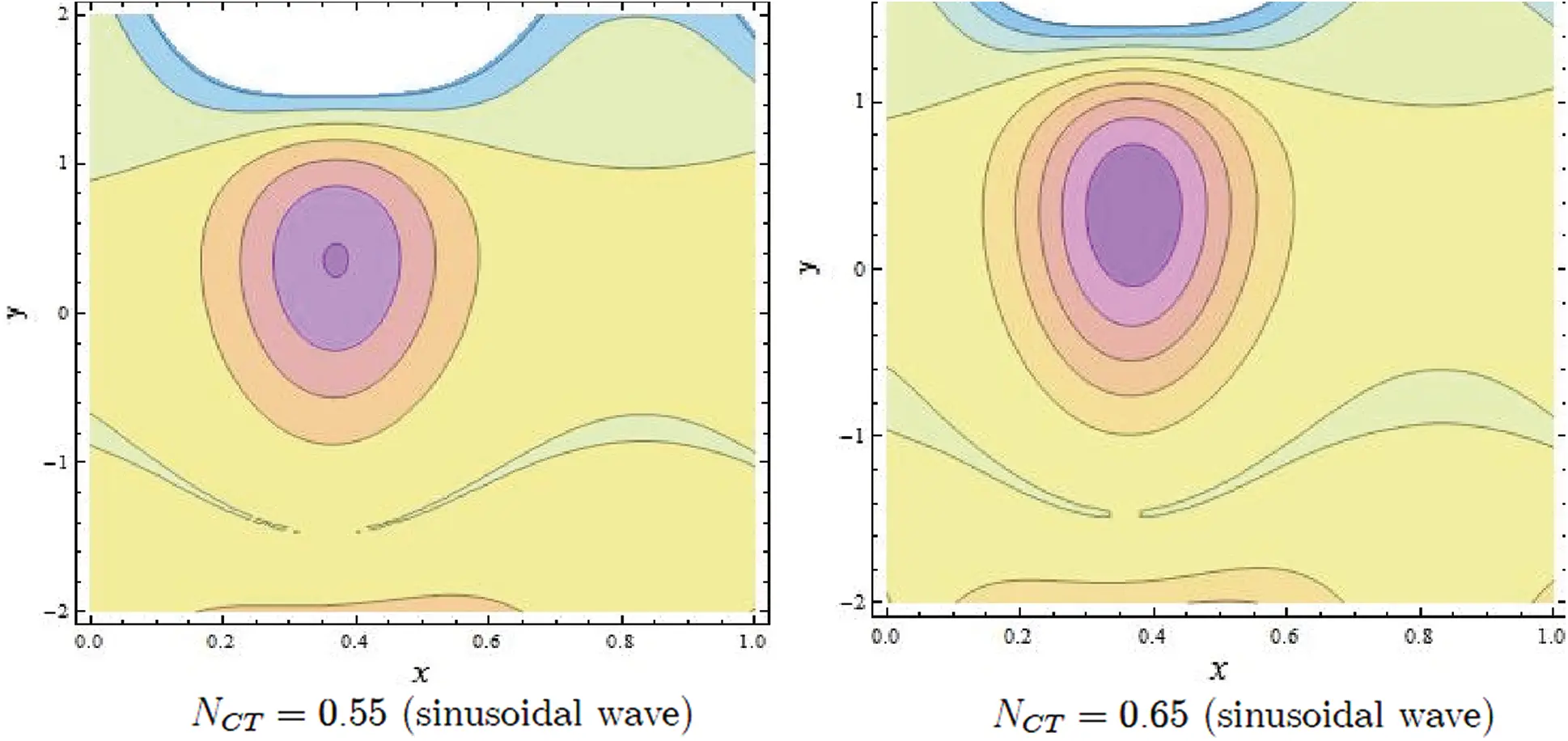Figure 8: Streamlines of NCT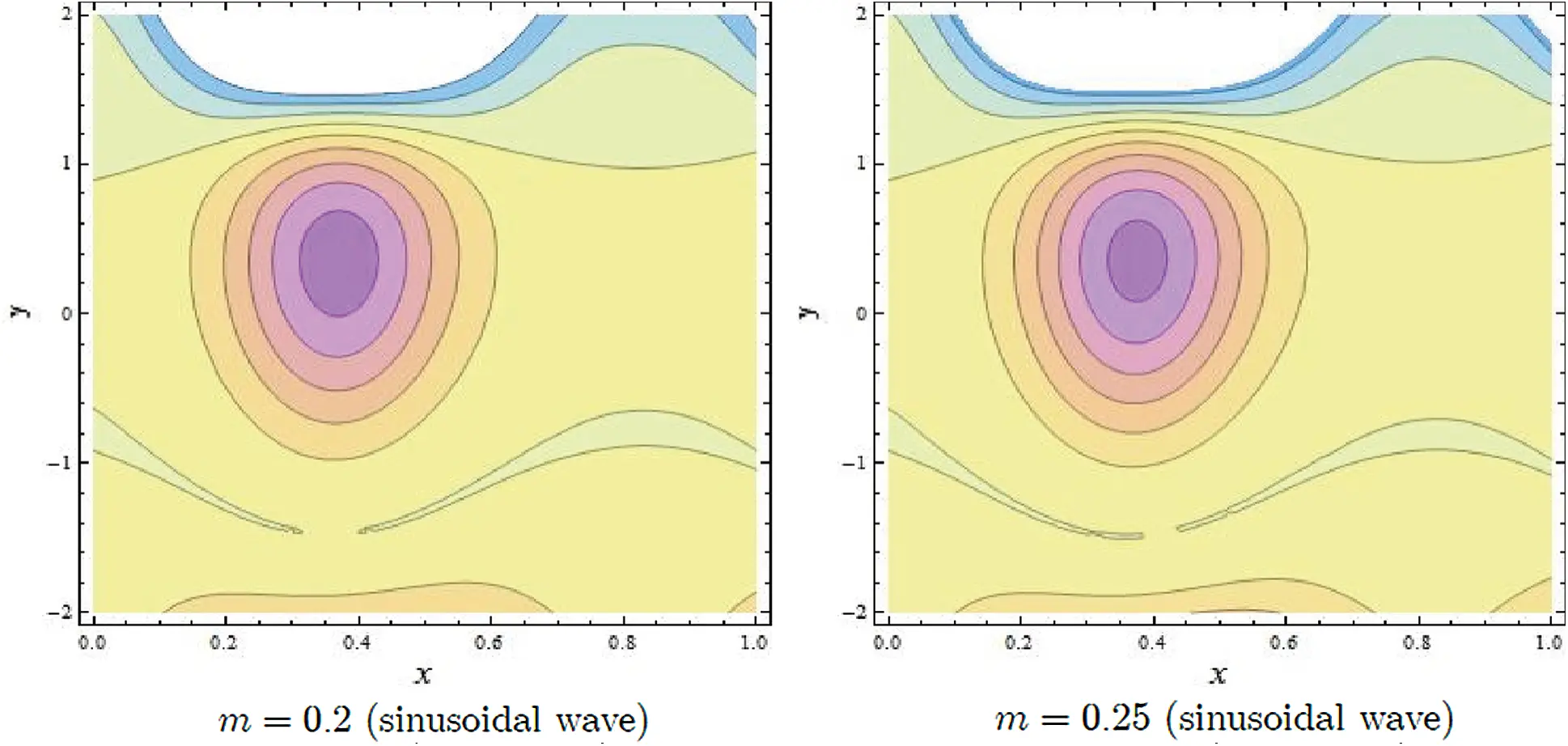Figure 9: Streamlines of m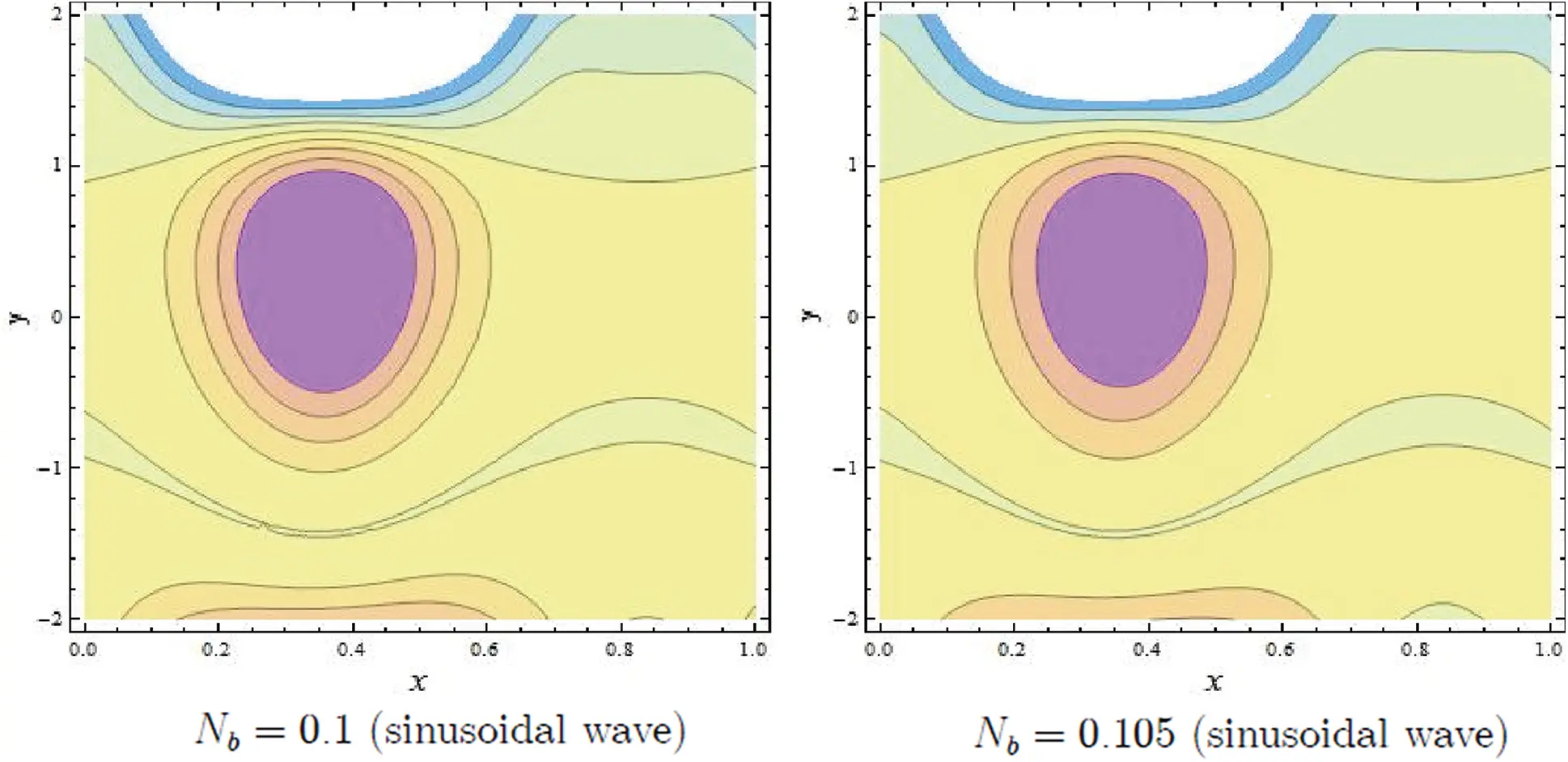Figure 10: Streamlines of Nb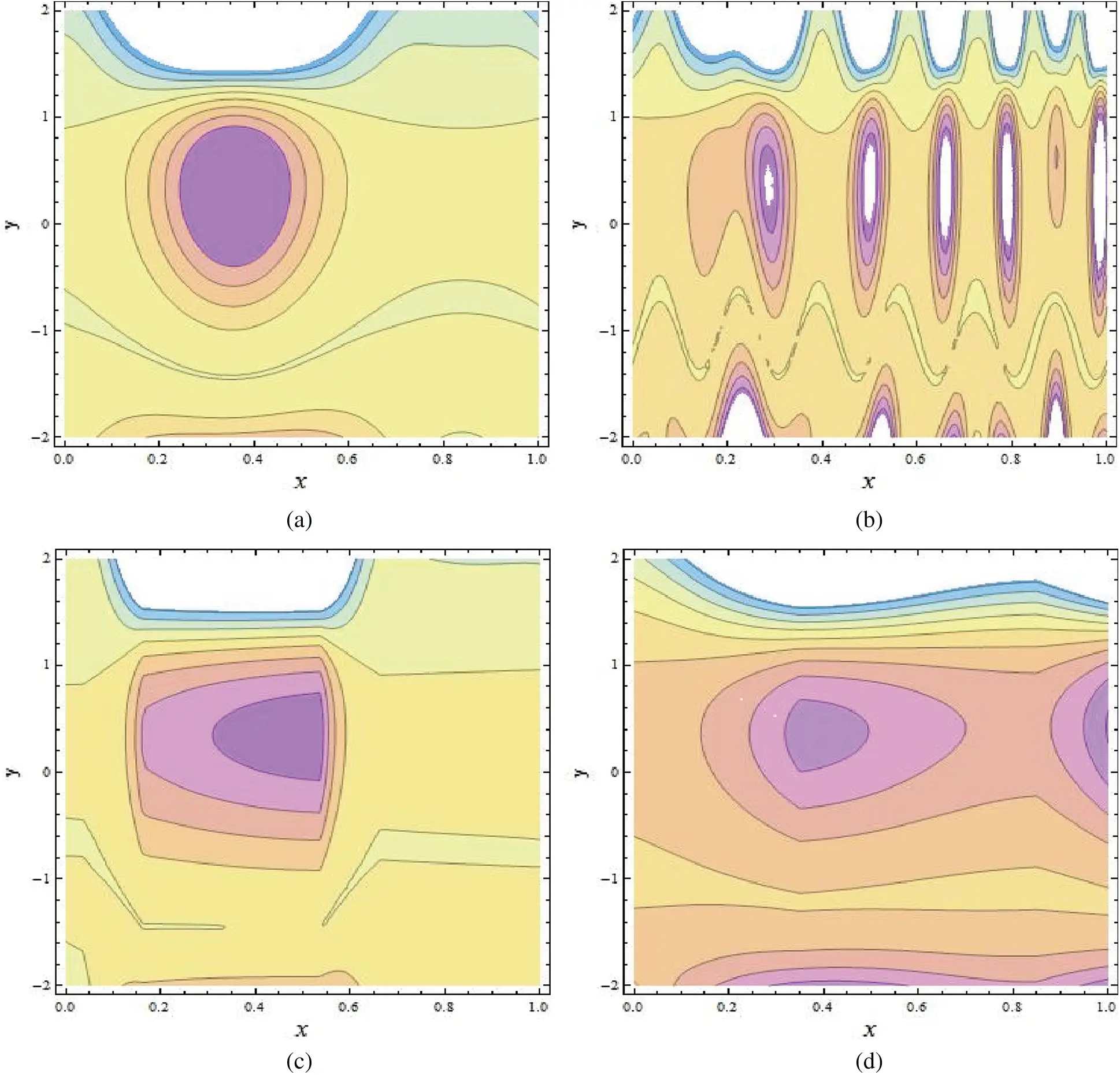Figure 11: (a–d): Streamlines of different wave shapes

7  Concluding Remarks

This article highlights the hybrid effects of thermal and concentration convection on peristaltic pumping of fourth grade nanofluids in an inclined tapered channel. The mathematical modelling of the fourth grade nanofluids is given along with thermal and concentration convection. Analytical technique is used to solve the differential equations that are strongly nonlinear in nature. Exact solutions of temperature, volume fraction of nanoparticles, and concentration are explored. The key finding can be encapsulated as follows:

•      The temperature profile behaviour decreases with increasing Nb values and increases with increasing NTC values.

•      The concentration profile increases with increasing Nt and NCT values.

•      The behaviour of nanoparticle fraction decreases because of the increasing values of Nb and NTC, whereas the behavior of nanoparticle fraction is quite opposite for Nt.

•      The behaviour of pressure gradient decreases due to the increasing values of Fr.

•      The size and number of the trapped bolus are increased by rising values of NCT and m.

Funding Statement: The authors received no specific funding for this study.

Conflicts of Interest: The authors declare that they have no conflicts of interest to report regarding the present study.

## References

1. Latham, T. W. (1966). Fluid motion in a peristaltic pump (Master Thesis). MIT, Cambridge.
2. Shapiro, A. H., Jaffrin, M. Y., & Weinberg, S. L. (1969). Peristaltic pumping with long wavelengths at low Reynolds number. Journal of Fluid Mechanics, 37, 799-825. [Google Scholar]
3. Fung, Y. C., & Yih, C. S. (1968). Peristaltic transport. Journal of Applied Mechanics, 35(4), 669-675. [Google Scholar] [CrossRef]
4. Bhatti, M. M., Zeeshan, A., Tripathi, D., & Ellahi, R. (2018). Thermally developed peristaltic propulsion of magnetic solid particles in Biorheological fluids. Indian Journal of Physics, 92(4), 423-430. [Google Scholar] [CrossRef]
5. Kothandapani, M., Pushparaj, V., & Prakash, J. (2018). Effect of magnetic field on peristaltic flow of a fourth-grade fluid in a tapered asymmetric channel. Journal of King Saud University–Engineering Sciences, 30(1), 86-95. [Google Scholar] [CrossRef]
6. Ellahi, R., Hussain, F., Ishtiaq, F., & Hussain, A. (2019). Peristaltic transport of Jeffrey fluid in a rectangular duct through a porous medium under the effect of partial slip: An application to upgrade industrial sieves/filters. Pramana, 93(3), 34. [Google Scholar] [CrossRef]
7. Akram, S., Mekheimer, K. S., & Abd Elmaboud, Y. (2018). Particulate suspension slip flow induced by peristaltic waves in a rectangular duct: Effect of lateral walls. Alexandria Engineering Journal, 57(1), 407-414. [Google Scholar] [CrossRef]
8. Riaz, A., Zeeshan, A., Ahmad, S., Razaq, A., & Zubair, M. (2019). Effects of external magnetic field on non-Newtonian two phase fluid in an annulus with peristaltic pumping. Journal of Magnetics, 24(1), 1-8. [Google Scholar] [CrossRef]
9. Haider, S., Ijaz, N., Zeeshan, A., & Li, Y. Z. (2019). Magneto-hydrodynamics of a solid-liquid two-phase fluid in rotating channel due to peristaltic wavy movement. International Journal of Numerical Methods for Heat & Fluid Flow, 30(5), 2501-2516. [Google Scholar] [CrossRef]
10. Riaz, A., Ellahi, R., Bhatti, M. M., & Marin, M. (2019). Study of heat and mass transfer in the Eyring–Powell model of fluid propagating peristaltically through a rectangular complaint channel. Heat Transfer Research, 16(16), 1539-1560. [Google Scholar] [CrossRef]
11. Eijkle, J. C. T., & van der Berg, A. (2005). Nanofluidics: What is it and what can we expect from it?. Microfluidics and Nanofluidics, 1(3), 249-267. [Google Scholar] [CrossRef]
12. Choi, S. U. S. (1995). Enhancing thermal conductivity of fluid with nanoparticles. Developments and applications of non-newtonian flows, 66, 99-105. [Google Scholar]
13. Masuda, H., Ebata, A., Teramae, K., & Hishinuma, N. (1993). Alteration of thermal conductivity and viscosity of liquid by dispersing ultra-fine particles. Netsu Bussei, 7(4), 227-233. [Google Scholar] [CrossRef]
14. Buongiorno, J., & Hu, W. (2005). Nanofluid coolants for advanced nuclear power plants. Proceedings of ICAPP: Vol. 5, pp. 15-19. Seoul.
15. Buongiorno, J. (2006). Convective transport in nanofluids. Journal of Heat Transfer, 128(3), 240-250. [Google Scholar] [CrossRef]
16. Kothandapani, M., & Prakash, J. (2015). Effect of radiation and magnetic field on peristaltic transport of nanofluids through a porous space in a tapered asymmetric channel. Journal of Magnetism and Magnetic Materials, 378(1), 152-163. [Google Scholar] [CrossRef]
17. Awais, M., Farooq, S., Hayat, T., & Ahmad, B. (2017). Comparative study of silver and copper water magneto nanoparticles with homogeneous-heterogeneous reactions in a tapered channel. International Journal of Heat and Mass Transfer, 115, 108-114. [Google Scholar] [CrossRef]
18. Prakash, J., Tripathi, D., Triwari, A. K., Sait, S. M., & Ellahi, R. (2019). Peristaltic pumping of nanofluids through tapered channel in porous environment: Applications in blood flow. Symmetry, 11(7), 868. [Google Scholar] [CrossRef]
19. Kothandapani, M., & Prakash, J. (2015). Effects of thermal radiation parameter and magnetic field on the peristaltic motion of Williamson nanofluids in a tapered asymmetric channel. International Journal of Heat and Mass Transfer, 81, 234-245. [Google Scholar] [CrossRef]
20. Ellahi, R., Zeeshan, A., Hussain, F., & Asadollahi, A. (2019). Peristaltic blood flow of couple stress fluid suspended with nanoparticles under the influence of chemical reaction and activation energy. Symmetry, 11(2), 276. [Google Scholar] [CrossRef]
21. Riaz, A., Khan, S. U., Zeeshan, A., Khan, S. U., & Hassan, M. (2020). Thermal analysis of peristaltic flow of nanosized particles within a curved channel with second-order partial slip and porous medium. Journal of Thermal Analysis and Calorimetry, 143(3), 1997-2009. [Google Scholar] [CrossRef]
22. Akram, S. (2016). Nanofluid effects on peristaltic transport of a fourth-grade fluid in the occurrence of inclined magnetic field. Scientia Iranica, 23(3), 1502-1516. [Google Scholar] [CrossRef]
23. Akbar, N. S. (2014). Metallic nanoparticles analysis for the peristaltic flow in an asymmetric channel with MHD. IEEE Transactions on Nanotechnology, 13(2), 357-361. [Google Scholar] [CrossRef]
24. Nadeem, S., Riaz, A., Ellahi, R., & Akbar, N. S. (2014). Mathematical model for the peristaltic flow of Jeffrey fluid with nanoparticles phenomenon through a rectangular duct. Applied Nanoscience, 4(5), 613-624. [Google Scholar] [CrossRef]
25. Zeeshan, A., Ellahi, R., Masood, F., & Hussain, F. (2019). Numerical study on bi-phase coupled stress fluid in the presence of hafnium and metallic nanoparticles over an inclined plane. International Journal of Numerical Methods for Heat and Fluid Flow, 29(8), 2854-2869. [Google Scholar] [CrossRef]
26. Ellahi, R., Raza, M., & Akbar, N. S. (2017). Study of peristaltic flow of nanofluid with entropy generation in a porous medium. Journal of Porous Media, 20(5), 461-478. [Google Scholar] [CrossRef]
27. Mosayebidorcheh, S., & Hatami, M. (2018). Analytical investigation of peristaltic nanofluid flow and heat transfer in an asymmetric wavy wall channel (Part I: Straight channel). International Journal of Heat and Mass Transfer, 126(3), 790-799. [Google Scholar] [CrossRef]
28. Hayat, T., Iqbal, R., Tanveer, A., & Alsaedi, A. (2016). Influence of convective conditions in radiative peristaltic flow of pseudoplastic nanofluid in a tapered asymmetric channel. Journal of Magnetism and Magnetic Materials, 408, 168-176. [Google Scholar] [CrossRef]
29. Hayat, T., Iqbal, R., Tanveer, A., & Alsaedi, A. (2016). Mixed convective peristaltic transport of Carreau–Yasuda nanofluid in a tapered asymmetric channel. Journal of Molecular Liquids, 223(2014), 1100-1113. [Google Scholar] [CrossRef]
30. Hussain, F., Ellahi, R., Zeeshan, A., & Vafai, K. (2018). Modelling study on heated couple stress fluid peristaltically conveying gold nanoparticle through coaxial tube: A remedy for gland tumor and arthritis. Journal of Molecular Liquids, 268, 149-155. [Google Scholar] [CrossRef]
31. Akbar, N. S. (2014). Double-diffusive natural convective peristaltic flow of a Jeffrey nanofluid in a porous channel. Heat Transfer Research, 45(4), 293-307. [Google Scholar] [CrossRef]
32. Asha, S. K., & Sunitha, G. (2020). Thermal radiation and hall effects on peristaltic blood flow with double diffusion in the presence of nanoparticles. Case Studies in Thermal Engineering, 17(4), 100560. [Google Scholar] [CrossRef]
33. Kuznetsov, A. V., & Nield, D. A. (2011). Double-diffusive natural convective boundary-layer flow of a nanofluid past a vertical plate. International Journal of Thermal Sciences, 50(5), 712-717. [Google Scholar] [CrossRef]
34. Akram, S., Razia, A., & Farkhanda, A. (2020). Effects of velocity second slip model and induced magnetic field on peristaltic transport of non-Newtonian fluid in the presence of double-diffusivity convection in nanofluids. Archive of Applied Mechanics, 90(7), 1583-1603. [Google Scholar] [CrossRef]
35. Sharma, A., Tripathi, D., Sharma, R. K., & Tiwari, A. K. (2019). Analysis of double diffusive convection in electroosmosis regulated peristaltic transport of nanofluids. Physica A, 535, 122148. [Google Scholar] [CrossRef]
36. Narayana, M., & Sibanda, P. (2012). On the solution of double-diffusive convective flow due to a cone by a linearization method. Journal of Applied Mathematics, 2012(5), 1-19. [Google Scholar] [CrossRef]
37. Nield, D. A., & Kuznetsov, A. V. (2011). The onset of double diffusive convection in a nanofluid layer. International Journal of Heat and Fluid Flow, 32(4), 771-776. [Google Scholar] [CrossRef]
38. Akram, S., Zafar, M., & Nadeem, S. (2018). Peristaltic transport of a Jeffrey fluid with double-diffusive convection in nanofluids in the presence of inclined magnetic field. International Journal of Geometric Methods in Modern Physics, 15(11), 1850181. [Google Scholar] [CrossRef]
39. Anwar Bég, O., & Tripathi, D. (2012). Mathematica simulation of peristaltic pumping with double-diffusive convection in nanofluids a bio-nanoengineering model. Proceedings of the Institution of Mechanical Engineers, Part N: Journal of Nanoengineering and Nanosystems, 225, 99-114. [Google Scholar]
40. Alolaiyan, H., Riaz, A., Razaq, A., Saleem, N., & Zeeshan, A. (2020). Effects of double diffusion convection on third grade nanofluid through a curved compliant peristaltic channel. Coatings, 10(2), 154. [Google Scholar] [CrossRef]

Appendix

ξ0=Nb(NCT+1)Grc-GrF(Nb+Nt)(h1-h2)Nb,ξ1=ξe(h1+h2)ξ(Nb(Grt-NCTGrc)+GrFNt)Nb(eh1ξ-eh2ξ),ξ2=24ξ12(ξ0-6L4ξ)ξ3,ξ3=-6ξ12(8ξ(L3ξ-3L4)+ξ0)ξ4,ξ4=12ξ0ξ1(ξ0-3L4ξ)ξ,ξ5=-6ξ1(36L42ξ2+2ξξ0(L3ξ-18L4)+3ξ02)ξ2,ξ6=-24ξ1(2L3ξ(3L4ξ-ξ0)+9L4(ξ0-4L4ξ))ξ2,ξ7=-24ξ1(L32ξ2+L3(ξ0-12L4ξ)+18L42)ξ2,ξ8=-24L3(L3ξ0+36L42),ξ9=-432L4(L3ξ0+6L42),ξ10=ξ3(2ξ2+ξξ3)-60ξ0ξ1216ξ8,ξ11=ξξ4-24ξ02ξ1ξ5,ξ12=ξ(12ξ4+ξξ5)-180ξ02ξ1ξ6,ξ13=ξ(60ξ4+ξ(8ξ5+ξξ6))-720ξ02ξ1ξ7,ξ14=ξ3ξ2-48ξ0ξ1216ξ7,ξ15=ξ7ξ4+4(30ξ4+ξ(5ξ5+ξξ6))ξ-1260ξ02ξ1ξ8,ξ16=h16ξ0ξ8240L3-2ξ13e-3h1ξ9ξ8+124h14(ξ8-36ξ02ξ1eh1(-ξ)ξ4),ξ17=h12(ξ12eh1(-ξ)-3ξ0ξ12e-2h1ξ4ξ6)+h1(ξ13eh1(-ξ)+ξ14e-2h1ξ),ξ18=1120h15ξ9+h13ξ11e-h1ξ+ξ10e-2h1ξ+ξ15e-h1ξ+ξ16+ξ17,ξ19=h26ξ0ξ8240L3-2ξ13e-3h2ξ9ξ8+124h24(ξ8-36ξ02ξ1e-h2ξξ4),ξ20=h22(ξ12e-h2ξ-3ξ0ξ12e-2h2ξ4ξ6)+h2(ξ13e-h2ξ+ξ14e-2h2ξ),ξ21=1120h25ξ9+h23ξ11e-h2ξ+ξ10e-2h2ξ+ξ15e-h2ξ+ξ19+ξ20,ξ22=16h13(ξ8-36ξ02ξ1e-h1ξξ4)+124h14ξ9-2ξξ10e-2h1ξ,ξ23=2h1(ξ12e-h1ξ-3ξ0ξ12e-2h1ξ4ξ6)+h13ξξ11(-e-h1ξ)+3h12ξ11e-h1ξ+ξ22,ξ24=h12(3ξ0ξ12e-2h1ξ2ξ5-ξξ12e-h1ξ)+ξ13e-h1ξ+ξ14e-2h1ξ+ξ23,ξ25=2ξ13e-3h1ξ3ξ7+3h14ξ02ξ1e-h1ξ2ξ3+h1(ξξ13(-e-h1ξ)-2ξξ14e-2h1ξ)-ξξ15e-h1ξ+ξ24,ξ26=16h23(ξ8-36ξ02ξ1e-h2ξξ4)+124h24ξ9-2ξξ10e-2h2ξ-ξξ15e-h2ξ,ξ27=2h2(ξ12e-h2ξ-3ξ0ξ12e-2h2ξ4ξ6)+h22(3ξ0ξ12e-2h2ξ2ξ5-ξξ12e-h2ξ),ξ28=3h24ξ02ξ1e-h2ξ2ξ3-h23ξξ11e-h2ξ+3h22ξ11e-h2ξ+ξ13e-h2ξ+ξ26+ξ27,ξ29=ξ14e-2h2ξ(2ξ13e-3h2ξ)3ξ7+h2(ξξ13(-e-h2ξ)-2ξξ14e-2h2ξ),ξ30=h12((h1-h2)3h22ξ4ξ0eh2ξ-24ξ1(h1(h2ξ+1)-h2(h2ξ+3))),ξ31=12h1ξ1e-h2ξ((h1-h2)(h1+2h2)ξ-6h2),ξ32=24ξ1e-h2ξ(-2h12ξ+h1(h2ξ+3)+h2(h2ξ+3)),ξ33=24ξ1(h12ξ+h1(h2ξ+3)+h2(3-2h2ξ)),ξ34=h13(-35h28L3ξ03-1440h27L3L4ξ02+1120h2L3ξ29+28h26ξ0ξ8ξ28-1120L3ξ21),ξ35=25h28L3ξ03+960h27L3L4ξ02+1120h2L3(ξ25-ξ29)-28h26ξ0ξ8ξ28+3360L3ξ21,ξ36=h2h12ξ35-1120h22h1L3(h2ξ25+3ξ18)+1120h23L3ξ18-28h23h16ξ0ξ8+ξ34,ξ37=5h22h18L3ξ02(7h2ξ0-192L4)+4h22h17ξ0(360h2L3L4ξ0+7ξ8)+ξ36,ξ38=3h12(h25ξ0(7ξ8ξ28-10h2L3ξ0(h2ξ0+42L4))280L3+ξ29),ξ39=h15ξ0(7ξ8-10h1L3ξ0(h1ξ0+42L4))280L3+ξ25,ξ40=ξ02(48(h17-h27)L4+(h18-h28)ξ0)-224(ξ18-ξ21),ξ41=25h28L3ξ03+960h27L3L4ξ02-28h26ξ0ξ8ξ28-3360L3(ξ18-ξ21),ξ42=h2(ξ41-1120h2L3(2ξ25+ξ29))-56h22h15ξ0ξ8+h1(ξ41+1120h2L3(ξ25-ξ29)),ξ43=4h17ξ0(20h2L3ξ0(h2ξ0-12L4)+7ξ8)+28h2h16ξ0(120h2L3L4ξ0+ξ8),ξ44=8h12(-10h27L3ξ03-420h26L3L4ξ02+7h25ξ0ξ8ξ28+140L3(ξ25+2ξ29)),ξ45=-15h28L3ξ03-600h27L3L4ξ02+560h2L3ξ25+560h2L3ξ29+14h2h15ξ0ξ8,ξ46=2h1(10h27L3ξ03+420h26L3L4ξ02-7h25ξ0ξ8ξ28-280L3(ξ25+ξ29)),ξ47=15h18L3ξ03-20h17L3ξ02(h2ξ0-30L4)+ξ45+14h26ξ0ξ8ξ28,L1=e-h2ξ(12F0(h1+h2)(h12-4h2h1+h22)ξ4eh2ξ+ξ30)+24h22ξ1e-h1ξ(h1(-h1ξ+h2ξ-3)+h2)24(h1-h2)3ξ4,L2=h2e-h1ξ(h1ξ4eh1ξ(72F0-(h1-h2)3(h1+h2)ξ0)-12ξ1(-2h12ξ+h1(h2ξ-6)+h22ξ))+ξ3112(h1-h2)3ξ4,L3=e-h1ξ(ξ4eh1ξ((h1-h2)3(h12+4h2h1+h22)ξ0-72F0(h1+h2))-ξ33)+ξ3224(h1-h2)3ξ4,L4=e-h1ξ(ξ4eh1ξ(24F0-(h1-h2)3(h1+h2)ξ0)+12ξ1(h1ξ-h2ξ+2))-ξ3412(h1-h2)3ξ4,L5=560F1(h1+h2)(h12-4h2h1+h22)L3-25h22h19L3ξ03+ξ371120(h1-h2)3L3,L6=-6h2(3224h12(-224F1+ξ40)-(h23-h13)ξ39)+(h1-h2)(h1+2h2)(ξ38-3h22ξ39)3h1(h1-h2)3,L7=-3360F1(h1+h2)L3-25h19L3ξ03-5h18L3ξ02(5h2ξ0+192L4)+ξ43+ξ44+ξ421120(h1-h2)3L3,L8=1120F1L3++1120L3ξ18-1120L3ξ21-14h16ξ0(60h2L3L4ξ0+ξ8)+ξ46+ξ47560(h1-h2)3L3,This work is licensed under a Creative Commons Attribution 4.0 International License, which permits unrestricted use, distribution, and reproduction in any medium, provided the original work is properly cited.